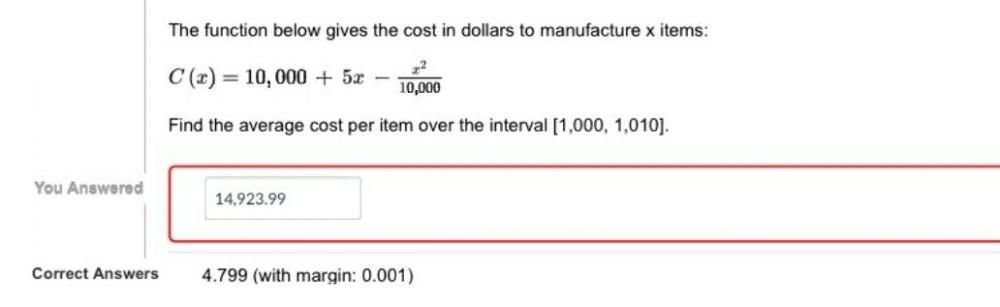Question:

# The function below gives the cost in dollars to manufacture x items: C (x) = 10,000 + 5x-x^2/10,000 Find the average cost per item over the interval [1,000, 1,010].The function below gives the cost in dollars to manufacture x items: C (x) = 10,000 + 5x-x^2/10,000 Find the average cost per item over the interval [1,000, 1,010].